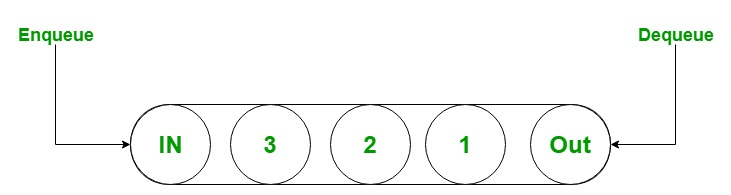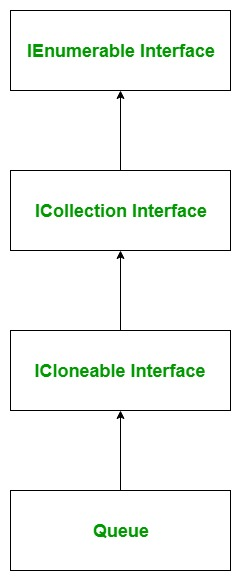Open in App
Not now

# C# Queue with Examples

• Difficulty Level : Easy
• Last Updated : 22 Feb, 2019

A Queue is used to represent a first-in, first out(FIFO) collection of objects. It is used when you need first-in, first-out access of items. It is the non-generic type of collection which is defined in `System.Collections` namespace. It is used to create a dynamic collection which grows, according to the need of your program. In Queue, you can store elements of the same type and of the different types. Generally, a queue is helpful when you access that information in the same way in which they stored in the collection and it is temporary storage to store data.The below diagram illustrates the Queue class hierarchy:Important Points:

• The Queue class implements the IEnumerable, ICollection, and ICloneable interfaces.
• When you add an item in the list, it is called enqueue.
• when you remove an item, it is called dequeue.
• Queue accepts null as a valid value for reference types.
• As elements are added to a Queue, the capacity is automatically increased as required by reallocating the internal array.
• In Queue, you are allowed to store duplicate elements.
• The capacity of a Queue is the number of elements the Queue can hold.

#### How to create the Queue?

Queue class has four constructors which are used to create the queue which are as follows:

• Queue(): This constructor is used to create an instance of Queue class which is empty and having the default initial capacity, and uses the default growth factor.
• Queue(ICollection): This constructor is used to create an instance of Queue class which contains elements copied from the specified collection, has the same initial capacity as the number of elements copied, and uses the default growth factor.
• Queue(Int32): This constructor is used to create an instance of Queue class which is empty and having specified initial capacity, and uses the default growth factor.
• Queue(Int32, Single): This constructor is used to create an instance of Queue class which is empty and having specified initial capacity, and uses the specified growth factor.

Let’s see how to create an Queue using Queue() constructor:

Step 1: Include System.Collections namespace in your program with the help of using keyword.

Syntax:

`using System.Collections;`

Step 2: Create an queue using Queue class as shown below:

`Queue queue_name = new Queue();`

Step 3: If you want to add elements in your queue then use Enqueue() method to add elements in your queue. As shown in the below example.

Example:

 `// C# program to illustrate queue ` `using` `System; ` `using` `System.Collections; ` ` `  `public` `class` `GFG { ` `    ``static` `public` `void` `Main() ` `    ``{ ` ` `  `        ``// Create a queue ` `        ``// Using Queue class ` `        ``Queue my_queue = ``new` `Queue(); ` ` `  `        ``// Adding elements in Queue ` `        ``// Using Enqueue() method ` `        ``my_queue.Enqueue(``"GFG"``); ` `        ``my_queue.Enqueue(1); ` `        ``my_queue.Enqueue(100); ` `        ``my_queue.Enqueue(``null``); ` `        ``my_queue.Enqueue(2.4); ` `        ``my_queue.Enqueue(``"Geeks123"``); ` ` `  `        ``// Accessing the elements ` `        ``// of my_queue Queue ` `        ``// Using foreach loop ` `        ``foreach``(``var` `ele ``in` `my_queue) ` `        ``{ ` `            ``Console.WriteLine(ele); ` `        ``} ` `    ``} ` `} `

Output:

```GFG
1
100

2.4
Geeks123
```

#### How to remove elements from the Queue?

In Queue, you are allowed to remove elements from the queue. The Queue class provides two different methods to remove elements and the methods are:

• Clear: This method is used to remove the objects from the queue.
• Dequeue: This method removes the beginning element of the queue.
• Example:

 `// C# program to illustrate how ` `// to remove elements from queue ` `using` `System; ` `using` `System.Collections; ` ` `  `public` `class` `GFG { ` `    ``static` `public` `void` `Main() ` `    ``{ ` ` `  `        ``// Create a queue ` `        ``// Using Queue class ` `        ``Queue my_queue = ``new` `Queue(); ` ` `  `        ``// Adding elements in Queue ` `        ``// Using Enqueue() method ` `        ``my_queue.Enqueue(``"GFG"``); ` `        ``my_queue.Enqueue(1); ` `        ``my_queue.Enqueue(100); ` `        ``my_queue.Enqueue(2.4); ` `        ``my_queue.Enqueue(``"Geeks123"``); ` ` `  `        ``Console.WriteLine(``"Total elements present in my_queue: {0}"``, ` `                                                    ``my_queue.Count); ` `        ``my_queue.Dequeue(); ` ` `  `        ``// After Dequeue method ` `        ``Console.WriteLine(``"Total elements present in my_queue: {0}"``, ` `                                                    ``my_queue.Count); ` ` `  `        ``// Remove all the elements from the queue ` `        ``my_queue.Clear(); ` ` `  `        ``// After Clear method ` `        ``Console.WriteLine(``"Total elements present in my_queue: {0}"``, ` `                                                    ``my_queue.Count); ` `    ``} ` `} `

Output:

```Total elements present in my_queue: 5
Total elements present in my_queue: 4
Total elements present in my_queue: 0
```

#### How to get topmost element of the queue?

In Queue, you can easily find the topmost element of the queue by using the following methods provided by the Queue class:

• Peek: This method returns the object at the beginning of the Queue without removing it.
• Dequeue: This method returns the object at the beginning of the Queue with modification means this method remove the topmost element of the queue.
• Example:

 `// C# program to illustrate how ` `// get topmost elements of the queue ` `using` `System; ` `using` `System.Collections; ` ` `  `public` `class` `GFG { ` `    ``static` `public` `void` `Main() ` `    ``{ ` ` `  `        ``// Create a queue ` `        ``// Using Queue class ` `        ``Queue my_queue = ``new` `Queue(); ` ` `  `        ``// Adding elements in Queue ` `        ``// Using Enqueue() method ` `        ``my_queue.Enqueue(``"GFG"``); ` `        ``my_queue.Enqueue(``"Geeks"``); ` `        ``my_queue.Enqueue(``"GeeksforGeeks"``); ` `        ``my_queue.Enqueue(``"geeks"``); ` `        ``my_queue.Enqueue(``"Geeks123"``); ` ` `  `        ``Console.WriteLine(``"Total elements present in my_queue: {0}"``, ` `                                                    ``my_queue.Count); ` ` `  `        ``// Obtain the topmost element of my_queue ` `        ``// Using Dequeue method ` `        ``Console.WriteLine(``"Topmost element of my_queue"` `                     ``+ ``" is: {0}"``, my_queue.Dequeue()); ` `                          `  ` `  `        ``Console.WriteLine(``"Total elements present in my_queue: {0}"``, ` `                                                    ``my_queue.Count); ` ` `  `        ``// Obtain the topmost element of my_queue ` `        ``// Using Peek method ` `        ``Console.WriteLine(``"Topmost element of my_queue is: {0}"``, ` `                                               ``my_queue.Peek()); ` ` `  `        ``Console.WriteLine(``"Total elements present in my_queue: {0}"``, ` `                                                    ``my_queue.Count); ` `    ``} ` `} `

Output:

```Total elements present in my_queue: 5
Topmost element of my_queue is: GFG
Total elements present in my_queue: 4
Topmost element of my_queue is: Geeks
Total elements present in my_queue: 4
```

#### How to check the availability of elements in the queue?

In Queue, you can check whether the given element is present or not using Contain() method. Or in other words, if you want to search an element in the given queue use Contains() method.

Example:

 `// C# program to illustrate how ` `// to check element present in ` `// the queue or not ` `using` `System; ` `using` `System.Collections; ` ` `  `class` `GFG { ` ` `  `    ``static` `public` `void` `Main() ` `    ``{ ` ` `  `        ``// Create a queue ` `        ``// Using Queue class ` `        ``Queue my_queue = ``new` `Queue(); ` ` `  `        ``// Adding elements in Queue ` `        ``// Using Enqueue() method ` `        ``my_queue.Enqueue(``"GFG"``); ` `        ``my_queue.Enqueue(``"Geeks"``); ` `        ``my_queue.Enqueue(``"GeeksforGeeks"``); ` `        ``my_queue.Enqueue(``"geeks"``); ` `        ``my_queue.Enqueue(``"Geeks123"``); ` ` `  `        ``// Checking if the element is ` `        ``// present in the Queue or not ` `        ``if` `(my_queue.Contains(``"GeeksforGeeks"``) == ``true``) { ` `            ``Console.WriteLine(``"Element available...!!"``); ` `        ``} ` `        ``else` `{ ` `            ``Console.WriteLine(``"Element not available...!!"``); ` `        ``} ` `    ``} ` `} `

Output:

```Element available...!!
```

#### Generic Queue Vs Non-Generic Queue

Generic Queue Non-Generic Queue
Generic queue is defined under System.Collections.Generic namespace. Non-Generic queue is defined under System.Collections namespace.
Generic queue can only store same type of elements. Non-Generic queue can store same type or different types of elements.
There is a need to define the type of the elements in the queue. There is no need to define the type of the elements in the queue.
It is type- safe. It is not type-safe.

My Personal Notes arrow_drop_up
Related Articles